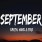# Typecho AJAX 利用 JS 无插件实现无刷新评论

``````    function ac() {
\$body = \$('html,body');
var g = '.comment-list',
h = '.comment-num',
j = '#textarea',
k = '',
l = '';
c();
\$('#comment-form').submit(function() {
\$.ajax({
url: \$(this).attr('action'),
type: 'post',
data: \$(this).serializeArray(),
error: function() {
return false
},
success: function(d) {
if (!\$(g, d).length) {
return false
} else {
k = \$(g, d).html().match(/id=\"?comment-\d+/g).join().match(/\d+/g).sort(function(a, b) {
return a - b
}).pop();
if (\$('.page-navigator .prev').length && l == "") {
k = ''
}
if (l) {
d = \$('#li-comment-' + k, d).hide();
if (\$('#' + l).find(".comment-children").length <= 0) {
\$('#' + l).append("<div class='comment-children'><ol class='comment-list'><\/ol><\/div>")
}
if (k) \$('#' + l + " .comment-children .comment-list").prepend(d);
l = ''
} else {
d = \$('#li-comment-' + k, d).hide();
"<h3>已有 <span class='comment-num'>0<\/span> 条评论<\/h3><ol class='comment-list'><\/ol>");
\$(g).prepend(d)
}
var f;
\$(h).length ? (f = parseInt(\$(h).text().match(/\d+/)), \$(h).html(\$(h).html().replace(f, f + 1))) : 0;
\$(j).val('');
c();
if (k) {
\$body.animate({
scrollTop: \$('#li-comment-' + k).offset().top - 50
}, 300)
} else {
\$body.animate({
}, 300)
}
}
}
});
return false
});

function c() {
\$(i + ',' + wi).click(function() {
l = \$(this).parent().parent().parent().attr("id")
});
l = ''
})
}
};
ac();
``````

``````<script src="//cdn.bootcss.com/jquery/2.1.4/jquery.min.js"></script>
<script src="<?php \$this->options->themeUrl('ajax.js'); ?>"></script>
``````

Ad：本站同款香港服务器 2H 1G 20G 400G 2M 最低只需要 ￥40 一月，地址： Pzea（xsx.net）

### 已有 8 条评论

1.塞梨Mac OS X 10.12.6Safari

这个博客也是无刷新评论吗

2.山卜方Mac OS XSafari

问下用了您的代码，回复的时候位置不在对应位置上，应该怎么改

1.站长 CyclistsMac OS XSafari

暂时无解，因为我发现有BUG，目前被困在老家，疫情还没结束，以后更新。

1.Veen ZhaoWindows 10Google Chrome

博主加油

1.站长 CyclistsWindows 7Google Chrome

谢谢

3.奶爸建网站笔记Windows 10Google Chrome

刷新一次不是还增加pv了。

1.站长 CyclistsWindows 7Google Chrome

随它，反正我个人已经不用任何统计了。